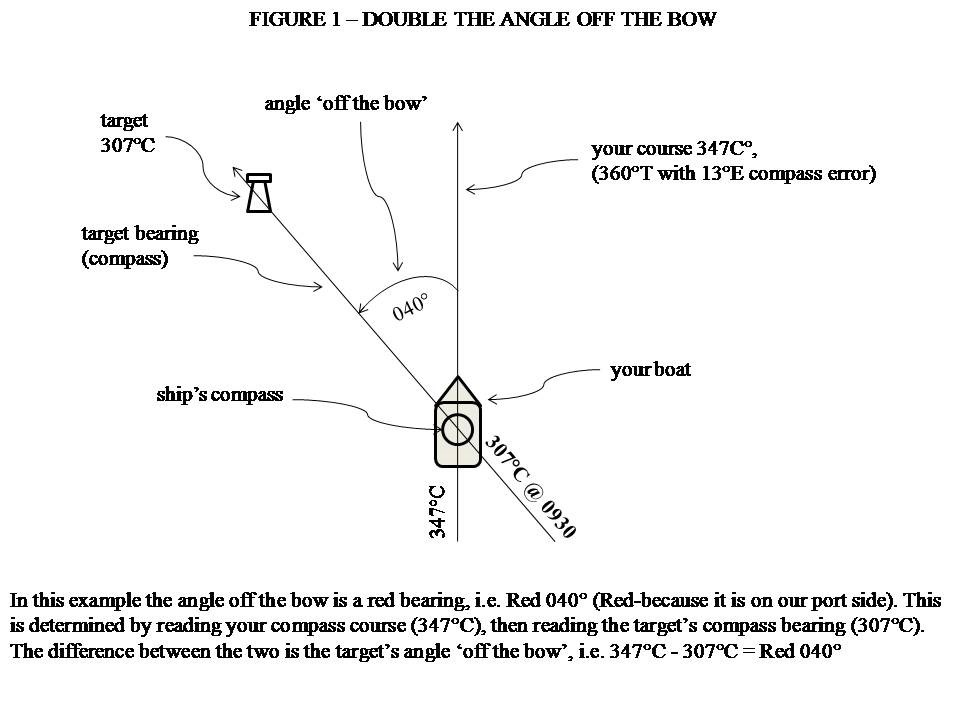# Double the Angle off your Bow

##### Finding your position with double the angle off the bow

This calculation takes advantage of the properties of an isosceles triangle. An isosceles triangle has two sides of equal length and consequently, it has two internal angles that are also equal. This rule is used when the second bearing between the bow of your boat and the target is double the angle of the first bearing.

###### How to perform ‘double the angle off the bow’ on board

See Figure 1(below) for a complete depiction of what double the angle off the bow refers to. Your first angle off the bow needs to be between 020° to 045° (less than 020° can reduce accuracy). So how do you measure this?

###### Step by step: how to calculate distance off, using double the angle off the bow method:1. Take note of the compass course you are steering. In our example, the course steered is 347°C.
2. With your hand-bearing compass, or by sighting over your ship’s compass, take a compass bearing of the chosen target (a lighthouse makes a good target).
3. Record this bearing, your log reading and the time. For example, Course = 347°C, bearing to Target (e.g. Nobby’s Head Lighthouse) = 307°C, Time = 0930hrs, Log reading = 0.
4. The difference between course steered and the target’s bearing is the target’s angle off the bow. Study Figures 1 and 2.

While steering a steady course of 347°C an identifiable land target (Nobby’s Head Lighthouse) was sighted bearing 307°C.

Angle off the bow = 347°C – 307°C = 040°

As the target is on our port side, we can call this angle RED 040°.

1. If our first angle off the bow = RED 040°, then doubling this angle will give us RED 080°.
2. The compass reading required for the second bearing line to the target will equal the course steered minus the double the angle off the bow.

See Figure 2.

347°C – 080° = 267°C

1. As you are proceeding along the chosen course (347°C), keep an eye on the target’s bearing. When the same target (Nobby’s Head Lighthouse) bears 267°C, note down (again) the time and distance run (from the log).

Course steered = 347°C, target bearing = 267°C, distance run = 5nm, time of second bearing = 1030hrs.

1. The number of sea miles covered (assuming no current or leeway) equals the distance the vessel is from the target. So you can say, ‘At 1030hrs we are 5nm from Nobby’s Head Lighthouse, which is on a bearing line of 267°C.’When you get the chance, have a go; it’s actually quite simple and straightforward.

Another useful method is to guess 045° off the bow using your arm. Swing your arm from pointing at the bow to pointing abeam of your boat. Half of this swing (090°/2) is 45°. When the target is 045° off the bow, note the time and log reading, double the angle off the bow will be 090° or abeam.

When target is abeam, note the time and log reading. As before, the distance run will equal your distance off the target.

This method is easier, but it is not predictive, i.e. you may have to avoid a danger that is lying off a headland. By using an initial angle off the bow less than 045° (e.g. 030°), you can predict your clearance off the headline on arrival. By using 045° as your initial angle, by the time 090° (abeam) comes up, you could be in the danger zone!

Remember: to be able to plot the fixed position on a chart, convert all bearings to TRUE. We have used a total Compass error for the above example of 13°E. So we have to add 13°E to all the Compass bearings to obtain the True bearing for the chart work.

### The Isosceles Triangle – how does it help us in Navigation?

Refer to Figure 2. A straight line (our course) = 180°. We cut that line with the double angle of 080°, therefore 180° – 080° = 100° remaining.

This 100° forms the angle at the top of our isosceles triangle.

All triangles have three internal corner angles. The value of all three angles added together always equals 180°.

In our example, we have 100°+ 40° = 140°. We know triangles have a total of 180° internally, therefore the remaining angle must = 40°. There it is: our isosceles triangle!

We have a baseline of a triangle, A to C and the two remaining sides (A to B and B to C) are equal in length because we have an isosceles triangle with two equal internal angles.

If we know the length of one side (our course covered, A to B) from our log reading, we then know the length of the side B to C.

A to B = 5 nautical miles, therefore B to C = 5 nautical miles.

We are 5 nautical miles from the target at 1030hrs.

===================================

What methods of positioning fixing would you like to learn about?